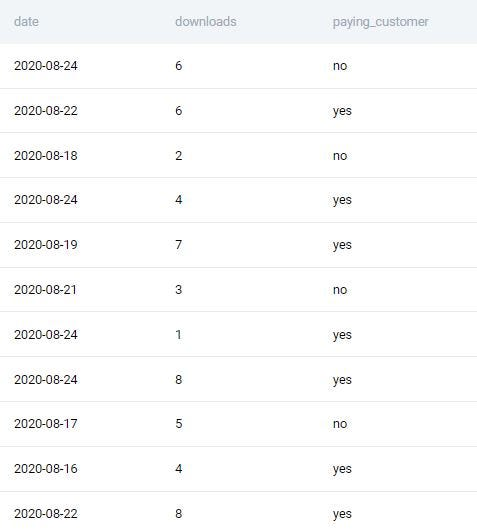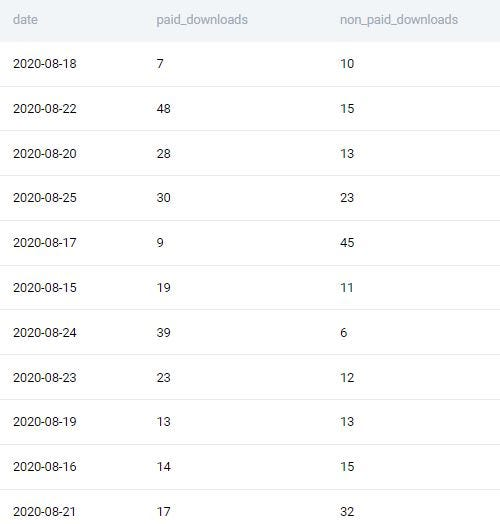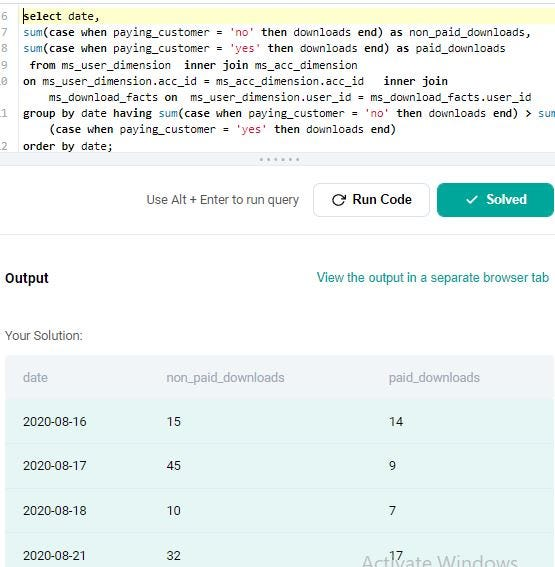R Gupta — Published On September 22, 2022

## Introduction

SQL proficiency is crucial for the field of data science. We’ll talk about two SQL queries that product businesses use to screen applicants for jobs as data scientists in this article. The StrataScratch website generates the SQL questions.

StataScratch is an excellent tool for anyone wishing to get started in data science and improve their SQL and Python skills. This platform offers coding questions and non-coding topics related to data science, such as statistics, probability, and so on. I strongly advise you to create an account on the StrataScratch website and practice the question along with the article. To solve this problem, I will use the Postgres SQL database.

If you know SQL well, you will stand a better chance of clearing data science interviews or dealing with day-to-day tasks efficiently. This article will focus on the approach to solving the problem. After going through this article, you would better understand how you should approach the solution for a given problem. You must read this article to improve your understanding and ways to approach solutions. Let’s get ahead to questions.

Interview Question Date: November, 2020, Company: Microsoft, Difficulty-Level: Medium, Interview QuestionsID: 10300, Tables: ms_user_dimension(fields: user_id(int), acc_id(int)), ms_acc_dimension (fields: acc_id(int), paying_customer(varchar)) , ms_download_facts(fields: date(datetime), user_id(int), downloads(int)

Preview of table ms_user_dimension:

Preview of table ms_acc_dimension:

Approach:

Three tables are provided here. To solve the problem, we must determine the number of daily downloads made by paying and nonpaying customers. The problem can be divided into three sections. We will join all of the tables in the first section. The second section will determine the number of paid and non-paid downloads for each user. Finally, we will show records with more non-paid downloads than paid downloads.

Step 1: Join TablesTo begin, we must combine the records from the various tables. As common field user_id exists between ms_user_dimension and ms_download_facts, and acc_id between ms_user_dimension and ms_acc_dimension. Therefore we can use an inner join to join all given tables and select the relevant columns date, downloads, and paying_customer columns which we need to shape the output. The code is given below for step1.

```select date, downloads,paying_customer from ms_user_dimension
```
```inner join ms_acc_dimension on
ms_user_dimension.acc_id = ms_acc_dimension.acc_id
``
```select date,
from ms_user_dimension  inner join ms_acc_dimension
on ms_user_dimension.acc_id = ms_acc_dimension.acc_id
group by date;```Step 3: Filter the Records and Format Result into Manner SpecifiedWe must display only those records in the result for which the number of paid_downloads exceeds non_paid downloads. paid_downloads and non_paid_downloads are calculated using the aggregation function. Therefore, we can not use them in the where clause. We can add a filter in having clause for fields calculated using aggregated function. And finally, sort the result such that the earliest date comes first. Here is the final code for the query:
```select date,
from ms_user_dimension  inner join ms_acc_dimension
on ms_user_dimension.acc_id = ms_acc_dimension.acc_id
group by date
having sum(case when paying_customer = 'no'
order by date;```In the preceding query, we saw how we could divide the problem into sections and work on each section individually. To answer the question, we used the join operation, case, group by, and having clause and how they are used to solve the question. Let’s work on the next query.

This is the hard-level question asked by Facebook/Meta in one of its interviews. You can view the question here. The question name is Highest Energy Consumption. The details of the question are given below:

#### Highest Energy Consumption

Find the date with the highest total energy consumption from the Meta/Facebook data centers. Output the date along with the total energy consumption across all data centers.

Interview Question Date: March 2020, Company: Meta/Facebook, Difficulty-Level: Medium, Interview QuestionsID: 10064, Tables: fb_eu_energy (field: date(datetime), consumption(int)), fb_asia_energy (field: date(datetime), consumption(int)) , fb_na_energy(field: date(datetime), consumption(int))

Preview of table fb_eu_energy:

Preview of table fb_asia_energy:

Preview of table fb_na_energy:

Approach: The problem will be divided into three sections. We will combine the records from the tables in the first section. The total energy consumed each day will be determined in the second part. Finally, we must determine the date on which the most energy was consumed and return the result.

Step 1: Join Tables

As data is present among three tables, we must combine all of the records from all three tables. We can’t use the union to combine records from these three tables because there are duplicate records in fb_eu_energy and fb_na_energy. For instance, the record (2020-01-01, 400) can be found in the fb_eu_energy and fb_na_energy tables. Therefore, if we combine records using the union, it will eliminate duplicate records. Therefore we have used union all, which will contain the duplicate record also.

```SELECT date, consumption
FROM  fb_eu_energy
union all
SELECT date, consumption FROM fb_asia_energy
union all
SELECT date, consumption FROM fb_na_energy;```

Step 2: Calculate the Total Amount of Energy Consumed for Each Day
After combining all records, we will select the sum of energy consumption for each day. We can use the group on the date and take the total energy consumed for this.

```select date, sum(consumption) as total_consumption
from (
SELECT date, consumption FROM fb_eu_energy
union all
SELECT date, consumption FROM fb_asia_energy
union all
SELECT date, consumption FROM fb_na_energy
)E
group by date;```

Step 3: Filter the Records and Format Result into Manner Specified

Now we must format our query result in the manner specified in the question. Across all data centers, we must output the data with the highest energy consumption. To arrange rows in descending order of total consumption, we can use the order by clause on the total consumption field. The first row will give us the date with the highest energy consumption; we can use limit 1 to output only one row for this task.

```select date, sum(consumption) as total_consumption from (
SELECT date, consumption FROM fb_eu_energy
union all
SELECT date, consumption FROM fb_asia_energy
union all
SELECT date, consumption FROM fb_na_energy
)E
group by date
order by sum(consumption)
desc limit 1 ;```

## Conclusion

In this article, we looked at two SQL questions and how to solve them efficiently. We have seen union all, group by, having clause, case clause, filtering the rows using where clause, and how they have been used to solve the questions. When attempting to solve any complex problem, keep the following points in mind

1. It is necessary to divide the problem into smaller problems. After reading the problem twice, decide which columns to use to calculate the desired result.
2. Next, determine the functions you’ll need to calculate each sub-problem, and then try to connect the solutions of the sub-problems.
3. Using this method, you can quickly solve any problem. Exposure to strategies for solving different questions by product companies will improve your capacity to formulate novel answers and new approaches to problems.